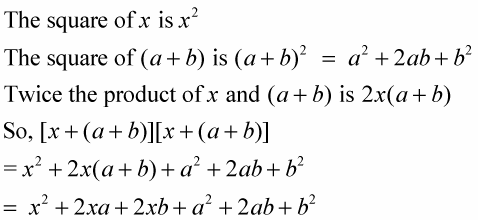##### Trigonometry Workbook For DummiesRecognizing a perfectly squared binomial can make life easier. When you recognize a perfectly squared binomial, you've identified a shortcut that saves time when distributing binomials over other terms.

When the same binomial is multiplied by itself — when each of the first two terms is distributed over the second and same terms — the resulting trinomial contains the squares of the two terms and twice their product. For example,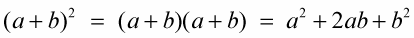Example 1: You can see with the following binomial that the same binomial is being multiplied by itself. So, the result of the distribution is the sum of the squares of x and 3 along with twice their product.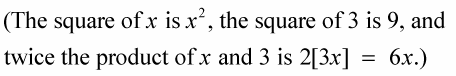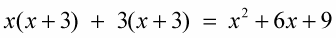Example 2: Try the binomial distribution, (4y – 5)(4y – 5), which contains negative signs.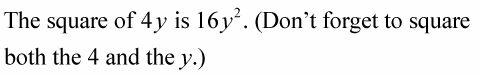The square of –5 is +25. (Note that the square is positive.)

Twice the product of 4y and –5 is 2(4y)(–5) = –40y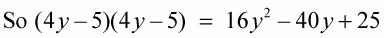Example 3: Use the shortcut for the expression,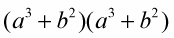where the terms are all variables.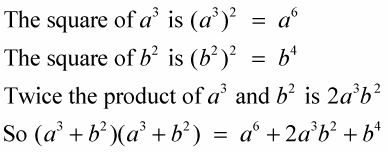Example 4: You can use the shortcut even with the expression, [x + (a + b)][x + (a + b)], where parentheses group the last two terms together in this distribution.